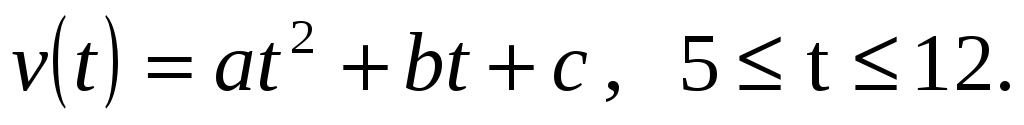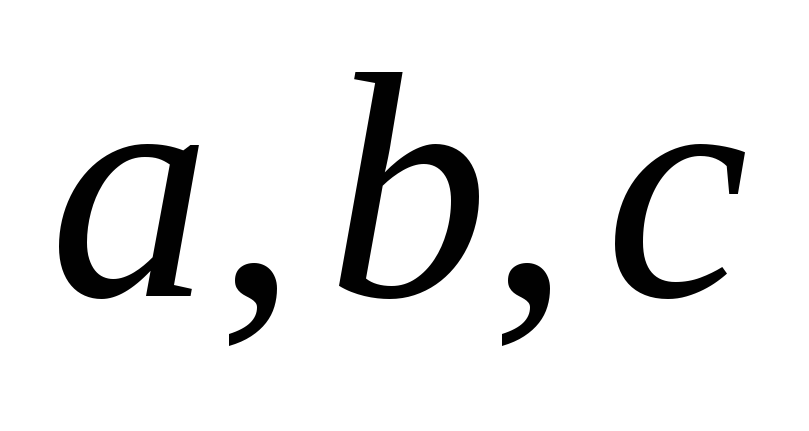# System of Equations 04. 05. Chapter 04. 05

 Page 1/87 Date 30.04.2018 Size 5.86 Mb. #47081

System of Equations 04.05.

###### System of Equations

After reading this chapter, you should be able to:

1. setup simultaneous linear equations in matrix form and vice-versa,

2. understand the concept of the inverse of a matrix,

3. know the difference between a consistent and inconsistent system of linear equations, and

4. learn that a system of linear equations can have a unique solution, no solution or infinite solutions.

## Matrix algebra is used for solving systems of equations. Can you illustrate this concept?

Matrix algebra is used to solve a system of simultaneous linear equations. In fact, for many mathematical procedures such as the solution to a set of nonlinear equations, interpolation, integration, and differential equations, the solutions reduce to a set of simultaneous linear equations. Let us illustrate with an example for interpolation.

## Example 1

The upward velocity of a rocket is given at three different times on the following table.

Table 5.1. Velocity vs. time data for a rocket
 Time, t Velocity, v (s) (m/s) 5 106.8 8 177.2 12 279.2

The velocity data is approximated by a polynomial asSet up the equations in matrix form to find the coefficientsof the velocity profile.